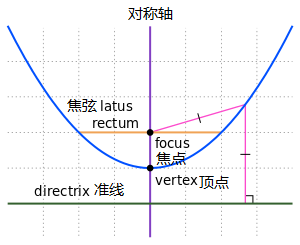(X-h)2 = 4a(Y-k)；  ( a = √(h-x1) * (h-x1) + (k - y1) * (k-y1) )

Y = (1/4a)X2 - (h/2a)X + (k + h2/4a)；( a = √(h-x1) * (h-x1) + (k - y1) * (k-y1) )